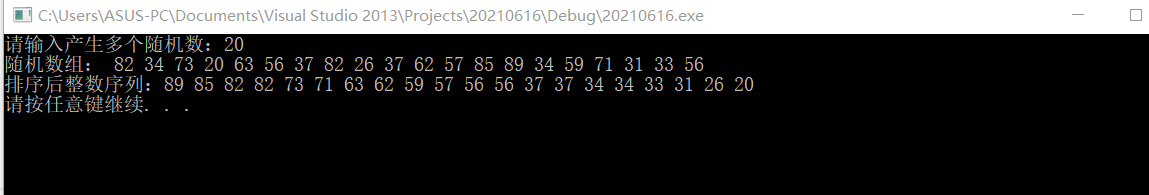# 写一个函数,对任意一维数组进行降序 排序。

• 写回答

#### 1条回答默认 最新

•抬头见山 2021-06-17 14:32
关注
``````#include <stdio.h>
#include <random>
#include <iostream>
#include <string>
#include <time.h>

using namespace std;

void InsertSort(int a[], int n)
{
for (int i = 1; i<n; i++) {
if (a[i] < a[i - 1]) {               //若第i个元素大于i-1元素，直接插入。小于的话，移动有序表后插入
int j = i - 1;
int x = a[i];        //复制为哨兵，即存储待排序元素
a[i] = a[i - 1];           //先后移一个元素
while (x < a[j]) {  //查找在有序表的插入位置
a[j + 1] = a[j];
j--;         //元素后移
}
a[j + 1] = x;      //插入到正确位置
}
}
}

int main()
{
cout << "请输入产生多个随机数：";
int ranNum,num = 0;
srand((unsigned)time(NULL));//time()用系统时间初始化种。为rand()生成不同的随机种子
cin >> num;
cout << "随机数组：";
for (int i = 0; i < num; i++)
{
ranNum[i] = 10 + (rand() % 80);
cout << " " << ranNum[i];
}
cout << endl;
InsertSort(ranNum, num);
cout << "排序后整数序列：";
for (int j = num-1; j >= 0; j--) {
cout << ranNum[j] << " ";
}
cout << endl;
system("pause");
return 0;
}``````本回答被题主选为最佳回答 , 对您是否有帮助呢?
评论

#### 悬赏问题

• ¥100 webapi的部署(标签-服务器)
• ¥20 怎么加快手机软件内部计时的时间(关键词-日期时间)
• ¥15 C语言除0问题的检测方法
• ¥15 为什么四分管的内径有的是16mm有的15mm，四分不应该是12.7mm吗
• ¥15 macos13下 ios交叉编译的问题
• ¥15 bgz压缩文件怎么打开
• ¥15 封装dll（引入了pcl的点云设计库）
• ¥30 关于#开发语言#的问题：我需要在抄板的基础上再抄板抄程序，根据RDA8851CM基础上开发
• ¥15 oracle 多个括号,怎么删除指定的括号及里面的内容?
• ¥15 小新14API2019想用bios调风扇